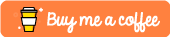Python Method Resolution Order Tutorial

Understanding the way and the order in which Python resolves functions is important. You need to know where your code is going to call, when you call it and things can get messy as soon as you start playing with classes inheriting from multiple other classes.

In this tutorial we’ll be looking at how Python 3 handles its MRO by using a little something called C3 Linearization.

The Problems with Inheritance

Imagine you were implementing a programming language that featured inheritance. When you first approach this topic you decide: a child class will have all of the functions and attributes that it’s parent classes should have!

Now this may work for the vast majority of scenarios but what would happen if two parent classes both implemented the same function or attribute? How do you decide what function or attribute takes precedence?

This is known as the diamond problem. Some languages such as Scala, use an algorithm called right-first depth-first search to solve this. Python 3 uses the C3 linearization algorithm.

A Pratical Example

Let’s take a look at how Method Resolution Order or MRO works in real-terms with a very simple Python program. We’ll define 2 classes that inherit nothing, and a third which inherits both.

What’s special about this is that the 2 classes our my_super_class class inherits from both define a test_func(self) function.

What happens when we run this code?

class awesome_class():

def __init__(self):
print("My Awesome Class")

def test_func(self):
print("This is my awesome class")

class not_so_awesome_class():

def __init__(self):
print("My Not So Awesome Class")

def test_func(self):
print("This is my not so awesome class")

class my_super_class(awesome_class, not_so_awesome_class):

def __init__(self):
print("My Super Class")

my_class = my_super_class()
my_class.test_func()

Output

When we run the above code you should see that the __init__ function is called of our my_super_class class. It then calls the inherited test_func(self) function from the its inherited awesome_class.

\$ python3.6 test.py
My Super Class
This is my awesome class

If we were to switch the order in which our my_super_class inherits then you’ll see that the test_func() from the not_so_awesome_class is called instead. Key takeaway from this point is that ordering of inheritance matters.

C3 Linearization

Let’s now have a look at this C3 superclass linearization algorithm and how it works. We can define it as so:

The C3 Superclass Linearization of a class is the sum of the class plus a unique merge of the linearizations of its parents and a list of the parents itself.

This may not mean a lot to most people, so let’s try and demystify what all this means by breaking it down.

C3 does the following:

• It guarantees that base class declaration is preserved
• It guarantees that subclasses appear before base classes
• It guarantees that for every class in a graph of all inherited classes, they adhere to the previous two points

An Example

Let’s have a look at the following class inheritance graph from the algo’s wiki:Basically in this graph class O does not have any parents and features a number of children: [A, B, C, D, E]. Our [A, B, C] classes are the parents to K1, [D, B, E] for K2 and [D, A] for K3. Finally class Z extends from [K1, K2, K3].

This represents a fairly complex dependency graph and not typically representative of “normal” systems, however it gives us lots of examples to dissect how C3 works.

Let’s start breaking this down:

L(O)This is relatively trivial and would be  as it has no parents
L(A)[A] + merge(l[O], [O])The linearization of A is A plus the merge of its parent’s,
[A, O]Thus the final linearization equals [A,O]
L(B)[B, O][B, C, D, E] all have very similar linearizations
L(K1)[K1] + merge(L(A), L(B), L©, [A,B,C])First we need to find the linearizations of K1’s parents, [A, B, C] and merge them with the parent list [A, B, C]
[K1] + merge([A,O], [B,O, [C,O], [A,B,C]])Class A is a good candidate as it is the head of the parent list
[K1, A] + merge([O], [B, O], [C, O], [B, C])We shouldn’t choose class O here as it appears in the tails of list 2 and 3, the next suitable class is B
[K1, A, B] + merge([O], [O], [C,O], [C])Again O is not a good candidate, we now go with C
[K1, A, B, C] + merge([O], [O], [O])Finally we have to choose class O as all other options are exhausted
[K1, A, B, C, O]Our final solution

Exercise: Work out the linearization of L(Z) based on examples above.

Conclusion

Hopefully this article gave you a little bit more insight into how Method Resolution Order works in Python 3. If you found this useful or require further assistance then please let me know in the comments section below.